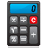﻿ 5. Detailed description of the Actions > 5.5. Standard > 5.5.1. Change Data Type (High-Speed action) > 5.5.1.3. Converting Numbers to Strings

# 5.5.1.3. Converting Numbers to Strings

Inside Anatella, there are basically two different notations to display floating-point numbers:

Standard notation.

Scientific notation.

“Short” notation (that is the shortest of the 2 above notations)

Here are some examples:

 Example 1 (a primary key) Example 2 (a small number) Example 3 (a large number) Example 4 (high precision number) Example 5 (procent or any number) Number 123456789 0.0000002 120000000 1-0.9-0.1 5.3 Standard notation limited to 16 chars (%.16f) 123456789 0.0000002 120000000 -0 5.3 Scientific notation limited to 16 chars (%.16e) 1.23456789E+08 2e-007 1.2e+008 -2.775557561562891e-017 5.3e+000 Shortest notation  limited to 16 chars (%.16g) 123456789 2e-007 1.2e+008 -2.775557561562891e-017 5.3 Standard notation using 6 chars to represent the fractional part (%f) 123456789.000000 0.000000 120000000.000000 -0.000000 5.300000 Scientific notation limited to 6 chars (%e) 1.234567e+008 2e-007 1.2e+008 -2.77556e-017 5.3e+000 Shortest notation  limited to 6 chars (%g) 1.234567e+008 2e-007 1.2e+008 -2.77556e-017 5.3 Standard notation limited to 5 chars (and 3 chars are used to represent the fractional part) (%5.3f) 1.23E+08 0.000 120000000.000 -0.000 5.300

The “shortest” notation is noted (%g). It’s based on either the standard (%f) or the scientific (%e) notation. The “shortest” notation is the more “compact” of the 2 basic notations (standard or scientific). Furthermore, trailing zeros are truncated, and the decimal point appears only if one or more digits follow it.

For the (%.16g): The scientific notation is used only when the exponent of the value is less than –4 or greater than 16.

For the (%g):        The scientific notation is used only when the exponent of the value is less than –4 or greater than 6.

To select a number notation follow these guidelines:

The easiest to read and the most compact notation is usually the (%g) notation.

This is the default notation that is used everywhere inside Anatella.

The (%g) notation is used:

oinside the data preview window.

oas default notation when exporting your data inside a simple text file.

oto (automatically) convert a number to a string inside theCalculator Action.

The (%g) notation works very well almost all the time. There however are 2 cases when it’s better to use the (%.16g) notation rather than the (%g) notation:

oWhen the number that you want to display is a primary key. In such case, you never want to use the scientific notation (especially for large numbers): See the example 1 here above.

oWhen you are exporting your data to another software for further processing, you don’t want any “rounding” or “truncation” errors that might jeopardize the next computations: See the example 4 here above.

When your numbers are “percents”, then it’s sometime better to use the (%5.3f) notation to obtain a nicer and clearer display.

If you want to use other notations inside Anatella, you can manually edit the .anatella files with a text editor and write the requested notation in it.

If you want more information about the different notations (%g,%e,%f) and the different precisions (%.16g or %5.3f), please refer to the following webpages: